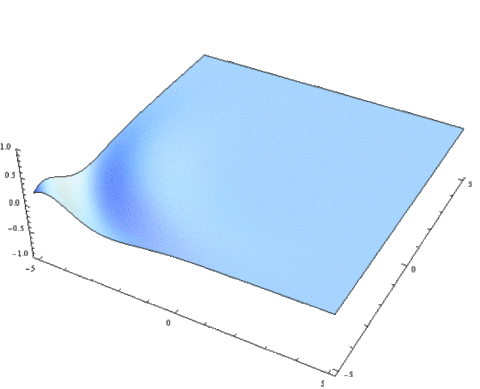Search

# Oscillation Oscillations - The beginning to studying waves (continuation)

Updated: Feb 21, 2020This post will include vocabulary and concepts which you can find explained in the previous post: Oscillations - The

beginning to studying waves Alright, this will end up being a short post, as I realized that much of what I wanted to cover was actually HL material and will come back in 9.1.

The graph you see below shows a displacement - time diagram. From these, you can read the period ( time taken for a complete oscillation, for example between to crests), amplitude (greatest distance to equilibrium position) and through calculation even the velocity, which is the gradient of the slope.While it is true that topic 4 doesn’t expect you to know the equations of the waves, it is good to know the idea. At equilibrium position (in this case t = 0), the velocity is at its max, and at max displacement, velocity is zero. If I would draw a velocity-time graph from the one above, it would look something like this:Following this logic, an acceleration-time graph looks like this:Oh, by the way, my experience when it comes to past exam questions tells me that a question like: Which of the following graphs shows simple harmonic motion?, often shows up. If that happens, the answer is usually the acceleration-displacement graph where a ∝ -xDue to the principle of conservation of energy (which I’ll hopefully cover in another post), we know that the energy within a system will remain constant. Therefore you can know that, in theory the energy of an oscillation will be the same. However, the form of energy will change during the oscillation.

Consider the following set-up:

At both A and B, the pendulum stops, if only for an instant, to change its direction. If you remember this, you can know that there is no kinetic energy at these position, and because, Total energy = Kinetic energy + Potential energy

Potential energy is at its max.

Likewise, at O there is zero displacement, hence zero potential energy, which gives max kinetic energy.

If you’ve read the previous post, you now (hopefully) know the entirety of 4.1, but don’t be afraid, there is still 4 subchapters to go before the topic is finished.